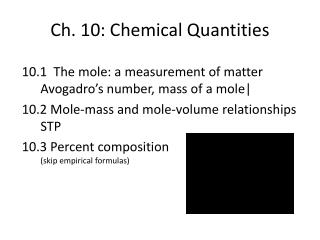DownloadDownload PresentationCh. 10: Chemical Quantities

# Ch. 10: Chemical Quantities

Download Presentation## Ch. 10: Chemical Quantities

- - - - - - - - - - - - - - - - - - - - - - - - - - - E N D - - - - - - - - - - - - - - - - - - - - - - - - - - -
##### Presentation Transcript

1. Ch. 10: Chemical Quantities 10.1 The mole: a measurement of matterAvogadro’s number, mass of a mole| 10.2 Mole-mass and mole-volume relationshipsSTP 10.3 Percent composition(skip empirical formulas)

2. 10.1 10.1 The Mole: A Measurement of Matter You could measure the amount of sand in a sand sculpture by counting each grain of sand, but it would be much easier to weigh the sand. You’ll discover how chemists measure the amount of a substance using a unit called a mole, which relates the number of particles to the mass.

3. 10.1 Measuring Matter What are three methods for measuring the amount of something? • You often measure the amount of something by one of three different methods—by count, by mass, and by volume.

4. Sample problem 10.1 Use dimensional analysis:

5. for Sample Problem 10.1 #1 on worksheet

6. 10.1 What is a Mole? • One mole (mol) of a substance is 6.02  1023 representative particles of that substance and is the SI unit for measuring the amount of a substance. • A mole of any substance contains Avogadro’s number of representative particles, or 6.02  1023 representative particles. • The term representative particle refers to the species present in a substance: usually atoms, molecules, or formula units.

7. 10.1 What is a Mole?

8. Sample problem 10.2

9. Sample Problem 10.2 #4 on worksheet On your calculator be sure to put the denominator in parentheses or divide by both 6.02 and 1023

10. Sample problem 10.3

11. 10.3

12. Sample Problem 10.3 #5 on worksheet

13. 10.1 The Mass of a Mole of an Element • The atomic mass of an element expressed in grams is the mass of a mole of the element. • The mass of a mole of an element is its molar mass. • Ex. On the periodic table, carbon has a mass of 12.011 amu. We say 12 grams per mole or just 12 grams.

14. 10.1 The Mass of a Mole of an Element One molar mass of carbon, sulfur, mercury, and iron are shown.

15. 10.1 The Mass of a Mole of an Element

16. 10.1 The Mass of a Mole of a Compound • To calculate the molar mass of a compound, find the number of grams of each element in one mole of the compound. Then add the masses of the elements in the compound. Substitute the unit grams for atomic mass units. Thus 1 mol of SO3 has a mass of 80.1 g.

17. 10.1 The Mass of a Mole of a Compound Molar Masses of Glucose, Water, and Paradichlorobenzene

18. Sample problem 10.4

19. Sample Problem 10.4 #7 on worksheet phosphorus molar mass = 30.974 gchlorine molar mass = 35.453 g 1 mole of phosphorus = 30.974 g3 mole of chlorine = 3 x 35.453 = 106.359 gmolar mass of PCl3 = 30.974 + 106.359 = 137 g/mol

20. 10.1 Section Quiz. 1. Three common ways of measuring the amount of something are by count, by mass, and a. by temperature. b. by volume. c. by area. d. by density.

21. 10.1 Section Quiz. 2. A mole of hydrogen gas, H2(g), contains 6.02 x 1023 a. molecules. b. atoms. c. amu. d. grams.

22. 10.1 Section Quiz. 3. The atomic mass of fluorine is 19.0 amu, so the molar mass is a. 19.0 amu. b. 19.0 g. c. 6.02 x 1023 amu. d. 6.02 x 1023 g.

23. 10.1 Section Quiz. 4. Calculate the molar mass of ammonium nitrate. a. 45.02 g b. 80.05 g c. 60.06 g d. 48.05 g NH4NO3 14+4(1)+14+3(16) g

24. 10.2 10.2 Mole–Mass and Mole–Volume Relationships How can you guess the number of jelly beans in a jar? You estimate the size of a jelly bean and then estimate the dimensions of the container to obtain its volume. In a similar way, chemists use the relationships between the mole and quantities such as mass, volume, and number of particles to solve chemistry problems.

25. 10.2 The Mole–Mass Relationship • How do you convert the mass of a substance to the number of moles of the substance? • Use the molar mass of an element or compound to convert between the mass of a substance and the moles of a substance.

26. Sample problem 10.5

27. Sample Problem 10.5 #9 on worksheet mass of carbon= 20 x 12.011 = 240.22 gmass of hydrogen = 42 x 1.008 = 42.336 gmass of C20H42 = 282.556 g/mol

28. Sample problem 10.6

29. Sample Problem 10.6 #11 on worksheet

30. 10.2 The Mole–Volume Relationship • Avogadro’s hypothesis states that equal volumes of gases at the same temperature and pressure contain equal numbers of particles. • Standard temperature and pressure (STP) means a temperature of 0°C or 273 K and a pressure of 101.3 kPa (kilopascals) or 1 atmosphere (atm). • The volume of a gas varies with temperature and pressure. Because of these variations, the volume of a gas is usually measured at a standard temperature and pressure.

31. 10.2 The Mole–Volume Relationship • At STP, 1 mol or, 6.02  1023 representative particles, of any gas occupies a volume of 22.4 L. • The quantity 22.4 L is called the molar volume of a gas.

32. 10.7

33. 10.7

34. Sample Problem 10.7 #13 on worksheet

35. 10.2 The Mole–Volume Relationship • Calculating Molar Mass from Density

36. Sample problem 10.8

37. Sample Problem 10.8 #15 on worksheet

38. 10.2 The Mole Road Map

39. 10.2 The Mole Road Map